# Selina Concise Mathematics Class 10 ICSE Solutions Chapter 25 Probability Ex 25C

## Selina Concise Mathematics Class 10 ICSE Solutions Chapter 25 Probability Ex 25C

These Solutions are part of Selina Concise Mathematics Class 10 ICSE Solutions. Here we have given Chapter 25 Probability Ex 25C.

Other Exercises

Question 1.
A bag contains 3 red balls, 4 blue balls and one yellow ball, all the balls being identical in shape and size. If a ball is taken out of the bag without looking into it : find the probability that die ball is :
(i) yellow
(ii) red
(iii) not yellow
(iv) neither,yellow nor red
Solution:
In a bag. there are 8 balls in which there are Red balls = 3
blue balls = 4
and yellow ball = 1
Total possible out come = 8
(i) Yellow balls = 1
∴ Number of favourable outcome = 1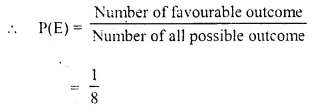(ii) Red balls = 3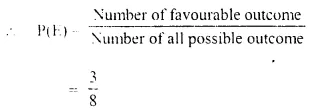(iii) Not yellow balls = 3+4 = 7
∴ Number of favourable outcome = 8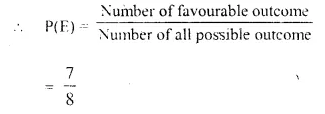(iv) Neither yellow nor red can be blue ball.
∴ Number of favourable outcome = 4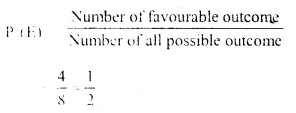Question 2.
A dice is thrown once. What is the probability of getting a number :
(i) greater than 2 ?
(iii) less than or equal to 2 ?
Solution:
A die is thrown once
Numbers marked on the faces of a die are 1, 2,3,4, 5, 6
∴ Number of possible outcome = 6
(i) Greater than 2 number = 3, 4, 5, 6 = 4 numbers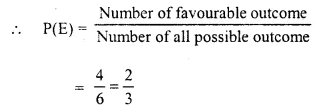(ii) Less than or equal to 2 are 2, 1 which are 2 in numbers.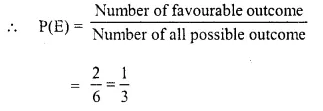Question 3.
From a well-shuffled deck of 52 cards* one card is drawn. Find the probability that the card drawn is :
(i) a face card.
(ii) not a face card. ,
(iii) a queen of black colour.
(iv) a card with number 5 or 6.
(v) a card with number less than 8.
(vi) a card with number between 2 and 9.
Solution:
A deck of playing cards has 52 cards
∴ Number of possible outcome = 52
(i) A face card ; face cards in the deck are = 3 x 4 = 12
∴ Number of favourable outcome = 12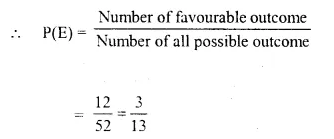(ii) Not a face card which are 52 – 12 = 40
∴ Number of favourable outcome = 40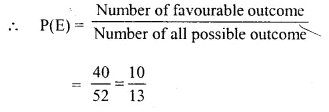(iii) A queen of black color which are 2 in numbers in the deck
∴ Number of favourable outcome = 2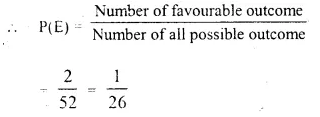(iv) A card with number 5 or 6 are 2 x 4 numbers
∴ Number of favourable outcome = 8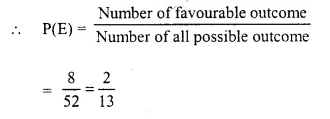(v) A card with number less than 8 which can be 2, 3, 4, 5, 6, 7 = 6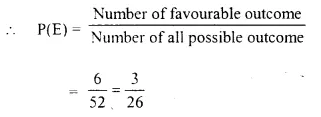(vi) A card with number between 2 and 9 can be 3, 4, 5, 6, 7, 8 = 6
∴ Number of favourable outcome = 6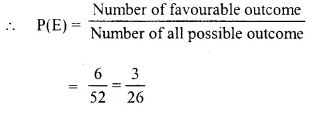Question 4.
In a match between A and B.
(i) the probability of winning of A is 0.83. What is the probability of winning of B ?
(ii) the probability of losing the match is 0.49 for
B. What is the probability of winning of A?
Solution:
A match is played between two persons A and B
∴ Number of possible outcome = 1
(i) The probability of winning of A is 0.83
∴ Probability of winning of B = 1 – 0.83 = 0.17 [∵ P(E) + P($$\bar { E }$$ )=1]
(ii) The probability of losing the match is 0.49 by B.
∴ Probability of losing of B or winning of A = 0.49

Question 5.
A and B are friends. Ignoring the leap year, find the probability that both friends will have :
(i) different birthdays ?
(ii) the same birthdays ? (Ignore a leap year)
Solution:
Number of days in a year = 365
and birthday of a person can be on one day only.
(i) Different birthdays can be 365 – 1 = 364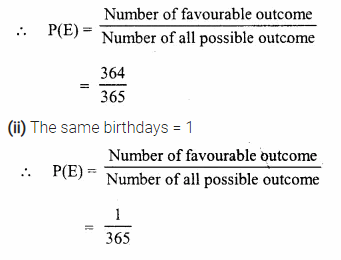Question 6.
A man tosses two different coins (one of Rs. 2 and another of Rs. 5) simultaneously. What is the probability that he gets :
(i) at least one head ?
Solution:
There are two coins : one of two rupees and
other is of 5-rupees
∴ Number of Heads =1 + 1=2
and number of tails are 2 i.e., 2 and 5
∴ Number of possible outcome = 2 x 2 = 4
Number of favourable outcome = 3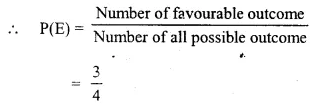(ii) At the most one head = 3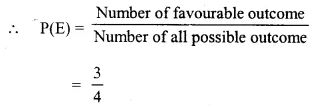Question 7.
A box contains 7 red balls, 8 green balls and 5 white balls. A ball is drawn at random from the box. Find the probability that the ball is :
(i) white
(ii) neither red nor white
Solution:
Number of balls in a box are 20 in which 7 are red, 8 are green and 5 are white
∴ Number of possible outcome = 20
(i) White = 5
∴ Number of favourable outcome = 5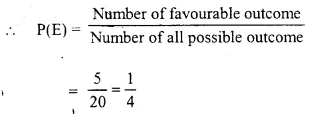(ii) Neither red not white
i.e., all the green which are 8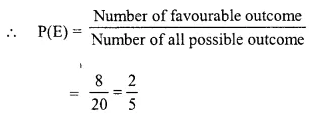Question 8.
All the three face cards of spades are removed from a well-shuffled pack of 52 cards. A card is then drawn at random from the remaining pack. Find the probability of getting :
(i) a black face card
(ii) a queen
(iii) a black card.
Solution:
A deck of playing cards = 52
Cards which are removed = 3 (3 face cards of spades)
Balance cards in the deck = 52 – 3 = 49
(i) A black face card which are 6 – 3 = 3 in number
∴ Number of favourable outcome = 3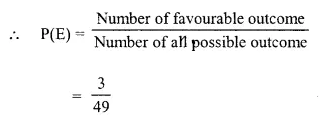(ii) A queen : In the deck there are 4 – 1 = 3 queen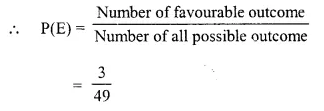(iii) A black cards : which are 26 – 3 = 23 cards in number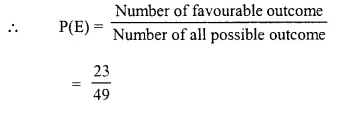Question 9.
In a musical chairs game, a person has been advised to stop playing the music at any time within 40 seconds after its start.
What is the probability that the music will stop within the first 15 seconds ?
Solution:
Total time for the musical race = 0 to 40 seconds = 40 seconds.
Time taken by a player =15 seconds. (0 to 15 seconds)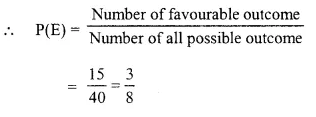Question 10.
In a bundle of 50 shirts, 44 are good, 4 have minor defects and 2 have major defects. What is the probability that :
(i) it is acceptable to a trader who accepts only a good shirt ?
(ii) it is acceptable to a trader who rejects only a shirt with major defects ?
Solution:
Total number of shirts in a bundle = 50
No. of good shirts = 44
Minor defected = 4
Major defected = 2
∴ Number of possible outcome = 50
(i) Acceptance of only for a good shirt = 44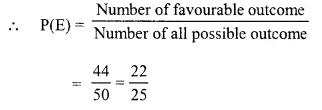(ii) Rejecting of totally defected shirts, number of remaining shirts = 44 + 4 = 48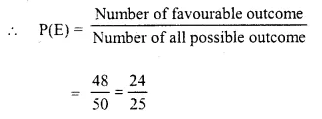Question 11.
Two dice are thrown at the same time. Find the probability that the sum of the two numbers appearing on the top of the dice is :
(i) 8
(ii) 13
(iii) less than or equal to 12
Solution:
Two dice are thrown at the same time and each dice has 6 numbers 1, 2, 3, 4, 5, 6 on its faces
∴Number of possible outcome = 6 x 6 = 36
(i) Sum of two numbers on the top is 8 i.e..(2, 6), (3, 5), (4,4), (5, 3), (6, 2)
∴ Number of favourable outcome = 5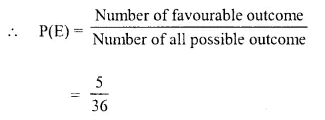(ii) Sum of two number on the top is 13.
At the most, then sum can be (6, 6) = 12
∴ number of favourable outcome = 0
∴ P(E) = 0
(iii) Sum is less than or equal to 12 i.e.(1, 1), (1,2), (1,3), (1,4), (1,5), (1,6), (2, 1), (2, 2), (2, 3), (2, 4), (2, 5), (2, 6), (3, 1), (3, 2), (3, 3). (3, 4), (3. 5), (3, 6), (4, 1), (4, 2), (4, 3), (4, 4), (4, 5), (4, 6), (5, 1), (5, 2), (5, 3), (5, 4), (5, 5), (5,
6) , (6, 1), (6, 2), (6, 3), (6, 4), (6, 5), (6, 6) = 36
∴ Number of favourable outcome = 36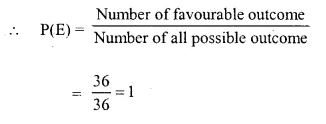Question 12.
Which of the following cannot be the probability of an event ?
(i) $$\frac { 3 }{ 7 }$$
(ii) 0.82
(iii) 37%
(iv) -2.4
Solution:
(i) ∵ $$\frac { 3 }{ 7 }$$ is between 0 and 1
∴ It is a probability event
(ii) ∵ 0.82 is between 0 and 1
∴ It is a probability event.
(iii) 37% = $$\frac { 37 }{ 100 }$$
∵It is between 0 and 1
∴ It is a probability event.
(iv) -2.4
∵It is less than 0.
∴ It is not a probability event.

Question 13.
If P(E) = 0.59; find P(not E).
Solution:
P(E) = 0.59
But P(E) + P($$\bar { E }$$ )= 1
or P(E) + P(not E) = 1
⇒ 0.59 + P(not E) = 1
⇒ ∴ P(not E) = 1 – 0.59 = 0.41

Question 14.
A bag contains a certain number of red balls. A ball is drawn. Find the probability that the ball drawn is :
(i) black
(ii) red.
Solution:
In a bag, there are certain number red balls. Let it be x balls.
One ball is drawn out
(i) A black
∵ There is no black ball in the bag
∴ Probability of black ball = 0
(ii) A red ball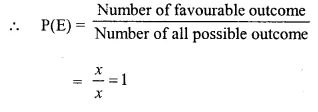Question 15.
The probability that two boys do not have the same birthday is 0.897. What is the probability that the two boys have the same birthday ?
Solution:
Probability of two boys do not have the same birthday [P (E)] = 0.897
Let Probability of those boys having the same birthday = P(not E)
= P($$\bar { E }$$ )
But P(E) + P($$\bar { E }$$ )= 1
⇒ 0.897 +P($$\bar { E }$$ ) = 1
⇒P($$\bar { E }$$ )= 1 -0.897 = 0.103
Hence probability having the same birthday = 0.103

Question 16.
A bag contains 10 red balls, 16 white balls and 8 green balls. A ball is drawn out of the bag at random. What is the probability tharthe ball drawn will be :
(i) not red ?
(ii) neither red nor green ?
(iii) white or green ?
Solution:
In a bag, there are 10 red balls, 16 white and 8 green balls
∴ Total balls =10 + 16 + 8= 34 ball
∴ Number of possible outcome = 34
(i) Not red ball
Number of favourable outcome = 16 + 8 = 24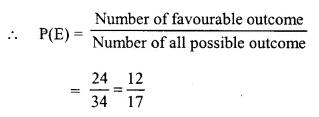(ii) Neither red nor green
∴ Number of outcome = 34 – (10 + 8)
= 34-18=16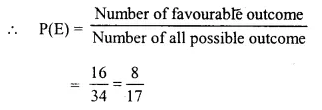(iii) White or green
∴ Number of outcome = 16 + 8 = 24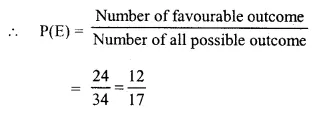Question 17.
A bag contains twenty Rs. 5 coins, fifty Rs. 2 coins and thirty Re 1 coins. If it is equally likely that one of the coins will fall down when the bag is turned upside down, what is the probability that the coin :
(i) will be a Re 1 coin ?
(ii) will not be a Rs. 2 coin ?
(iii) will neither be a Rs. 5 coin nor be a Re 1 coin ?
Solution:
In a bag, there are
5-rupee coins = 20
2-rupee coins = 50
1-rupee coin =30
Total number of coins in the bag = 20 + 50 + 30 = 100
∴ Number of possible outcome =100
(i) one-rupee coin = 30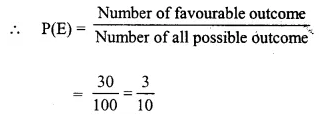(ii) When there is no 2-rupee coins
∴ Number of coins = 20 + 30 = 50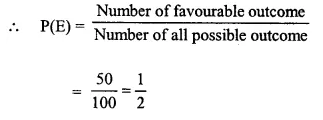(iii) Neither be Rs. 5 coins nor be Re-one coin = 100 – (20 + 30) = 100 – 50 = 50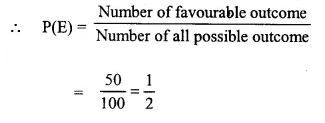Question 18.
A game consists of spinning an arrow which comes to rest pointing at one of the numbers 1, 2, 3, 4, 5, 6, 7, 8, 9, 10, 11, 12; as shown below.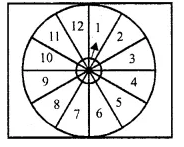If the outcomes are equally likely, find the probability that the pointer will point at :
(i) 6
(ii) an eve., number.
(iii) a prime number.
(iv) a number greater than 8.
(v) a number less than or equal to 9.
(vi) a number between 3 and 11.
Solution:
There are 12 numbers on the spinning game.
∴ Number of possible outcome = 12
(i) 6 which is one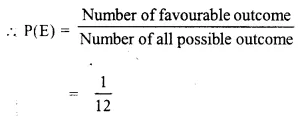(ii) an even number which are 6 i.e. 2, 4, 6, 8, 10, 12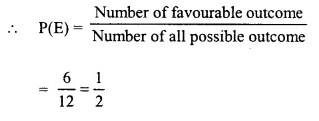(iii) A prime number which are 2, 3, 5, 7, 11 and 5 in number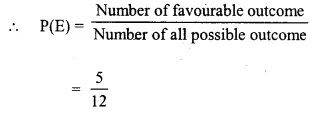(iv) A number greater than 8 are 9, 10, 11, 12
which are 4 in number.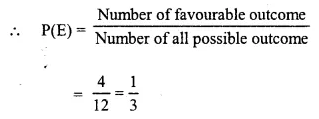(v) A number less than or equal to 9 are
1, 2, 3, 4, 5, 6, 7, 8, 9 which are 9 in number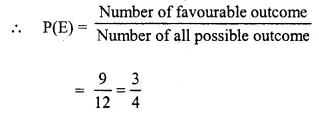(vi) A number between 3 and 11 are 4, 5, 6, 7, 8, 9, 10 which are 7 in number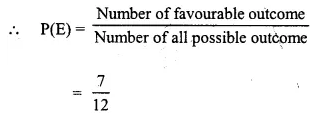Question 19.
One card is drawn from a well-shuffled deck of 52 cards. Find the probability of getting :
(i) a queen of red colour.
(ii) a black face card.
(iii) the jack or the queen of hearts.
(iv) a diamond.
(v) a diamond or a spade.
Solution:
Number of cards in a deck of playing card = 52
∴ Number of possible outcome = 52
(i) A queen of red colour
Number of favourable outcome = 2
(As there are 2 red queens in the deck)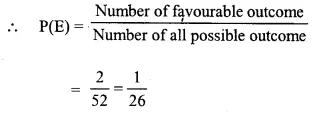(ii) A black face card.
There are 3 + 3 = 6 black face cards in the deck.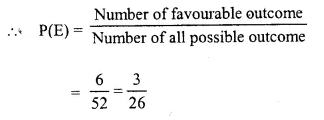(iii) The jack or the queen of hearts which are two in numbers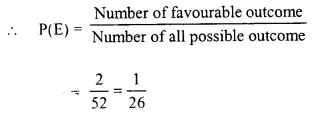(iv) A diamond.
There are 13 cards of diamond in the deck.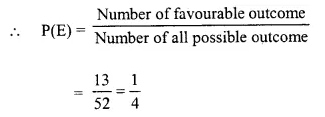(v) A diamond or a spade.
There are 13 cards of diamond and 13 cards of spade in the deck
∴ Number of favourable outcome = 13 + 13 = 26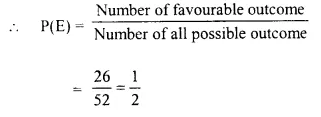Question 20.
From a deck of 52 cards, all the face cards are removed and then the remaining cards are shuffled. Now one card is drawn from the remaining deck. Find the probability that the card drawn is :
(i) a black card.
(ii) 8 of red color.
(iii) a king of black color.
Solution:
Number of cards is a deck of playing card = 52
All face cards are removed. Which are 3 x 4 = 12
(i) A black card which are 10 in numbers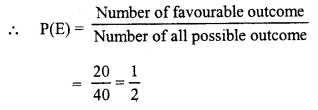(ii) 8 of red colors(iii) A king of black color.
∵ In the deck of playing cards, face cards are removed
∴ There is no face cards.
∴ P(E) = 0.

Question 21.
Seven cards : – the eight, the nine, the ten, jack, queen, king and ace of diamonds are well shuffled. One card is then picked up at random.
(i) What is the probability that the card drawn is the eight or the king ?
(ii) If the king is drawn and put aside, what is the probability that the second card picked up is
(a) an ace ?
(b) a king ?
Solution:
There are 7 cards which are the eight, the nine, the ten, the jack, the’ queen, the king and the ace of diamond.
∴ Number of possible outcome = 7
(i) card having the eight or a king which are 2.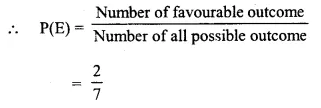(ii) If king is drawn, then number of remaining playing cards = 7-1=6
(a) An ace.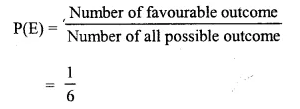(b) A king
There is no card of king
∴ P(E) = 0

Question 22.
A box contains 150 bulbs out of which 15 are defective. It is not possible to just look at a bulb and tell whether or not it is defective. One bulb is taken out at random from this box. Calculate the probability that the bulb taken out is :
(i) a good one
(ii) a defective one.
Solution:
Number of bulbs in a box = 150
No. of defective bulbs = 15
∴ No of good bulbs = 150 – 15 = 135
∴ Number of possible outcome = 150
(i) A good bulb and number of good bulbs =135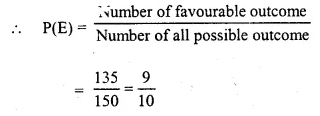(ii) A defective bulb. Number of defective bulb = 15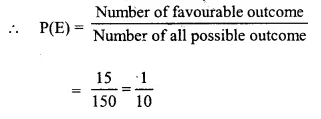Question 23.
(i) 4 defective pens are accidentally mixed with 16 good ones. It is not possible to just look at a pen and tell whether or not it is defective. One pen is drawn at random from the lot. What is the probability that the pen is defective ? (ii) Suppose the pen drawn in (i) is defective and is not replaced. Now one more pen is drawn at random from the rest. What is the probability that this pen is :
(a) defective
(b) not defective ?
Solution:
Number of defective pens = 4
(i) and number of good pens = 16
∴ Total pens = 4 + 16 = 20
∴ Number of possible outcome = 20
One defective pen, no. of defective pens = 4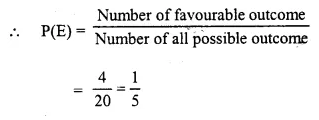(ii) One defective pen is drawn
∴ Remaining pens = 20 – 1 = 19
(a) Defective one
Number of remaining defective pens =4-1=3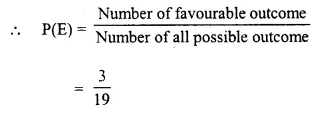(b) Not defective, number of good pens =16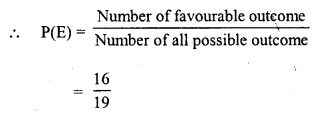Question 24.
A bag contains 100 identical marble stones which are numbered from 1 to 100. If one stone is drawn at random from the bag, find the probability that it bears :
(i) a perfect square number.
(ii) a number divisible by 4.
(iii) a number divisible by 5.
(iv) a number divisible by 4 or 5.
(v) a number divisible by 4 and 5.
Solution:
Total number of stones = 100
On which numbers 1 to 100 are marked
∴ Number of possible outcome = 100
(i) A perfect square = which are 1, 4, 9, 16, 25, 36, 49, 64, 81, 100 which are 10 inmumbers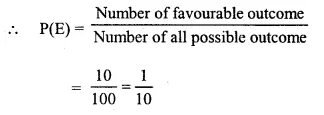(ii) A number divisible by 4 are 4, 8, 12, 16, …, 96, 100 which one 25 in numbers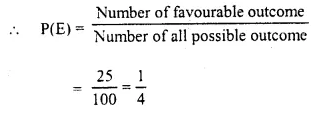(iii) A number divisible by 5, are 5, 10, 15, 20, ….., 95, 100 which are 20 in numbers,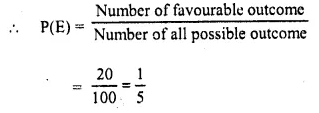(iv) A number divisible by 4 or 5 are 4, 5, 8, 10,12, 15, 16, 20, 24, 25, 28, 30, 32, 35, 36, 40, 96,95, 100 which are 40 in numbers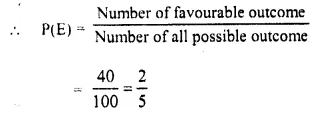(v) A number divisible by 4 and 5 are 20, 40, 60, 80 and 100 which are 5 in numbers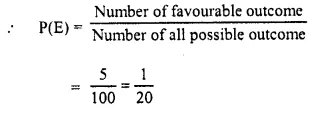Question 25.
A circle with diameter 20 cm is drawn somewhere on a rectangular piece of paper with length 40 cm and width 30 cm. This paper is kept horizontal on table top and a die, very small in size, is dropped on the rectangular paper without seeing towards it. If the die falls and lands on the paper only, find the probability that it will fall and land :
(i) inside the circle.
(ii) outside the circle.
Solution:
Diameter of the circle = 20 cm
Length of rectangular paper = 40 cm
and width = 30 cm
Area of rectangle = 40 x 30 = 1200 cm2
∴ Number of possible out come = 1200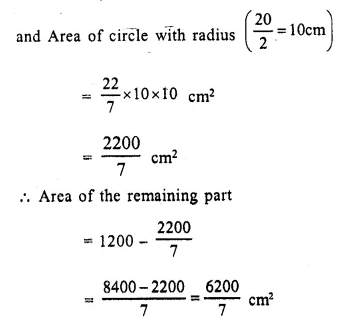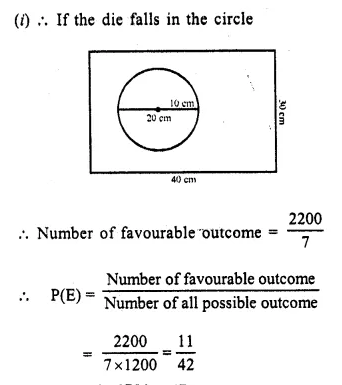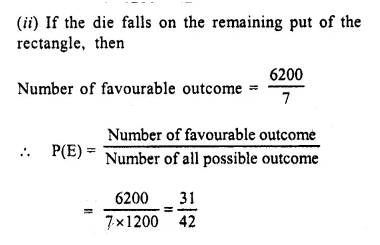Question 26.
Two dice (each bearing numbers 1 to 6) are rolled together. Find the probability that the sum of the numbers on the upper-most faces of two dice is :
(i) 4 or 5
(ii) 7, 8 or 9.
(iii) between 5 and 8
(iv) more than 10
(v) less than 6
Solution:
Two dice having numbers 1 to 6 are rolled together.
∴ Number of possible outcome = 6 x 6 = 36
(i) If sum is 4 or 5 of numbers on the upper most face,
Their number of favourable outcome = (1,3), (2, 2), (3, 1), (1, 4), (2, 3), (3, 2), (4, 1) which are 7 in numbers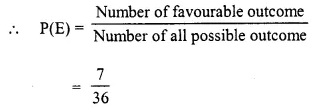(ii) If sum of number on the upper faces by 7, 8 or 9, then these can be (1, 6), (2, 6), (3, 6), (4, 3), (5. 2). (6, 1). (4, 3), (2, 5), (3, 4), (4, 4), (5, 3), (5, 4), (6, 2), (6, 3), (4, 5) which are total 15 in numbers.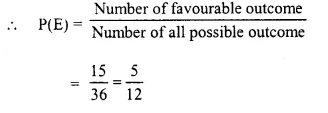(iii) Sum is between 5 and 8.
i.e. sum is 6 or 7.
These can be.
(1, 5), (2, 5), (1, 6), (5, 1), (5, 2), (6, 1), (3, 3), (3, 4), (4, 3), (2, 4), (4, 2) which are 11 in number.
∴ Number of favourable outcome = 11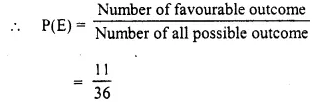(iv) If sum is more than 10, then these can be (5. 6), (6, 5), (6, 6) which are 3 in number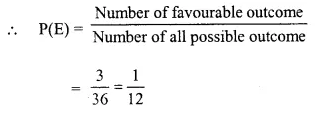(v) If sum is less than 6, then there can be (1, 2), (2, 1), (1, 3), (3, 1), (4, 1), (1, 4), (2, 3), (3, 2), (2, 4), (4, 2)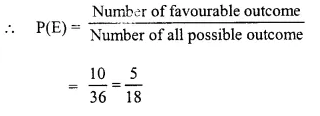Question 27.
Three coins are tossed together. Write all the possible outcomes. Now, find the probability of getting :
(iv) all tails.
(v) at least one tail.
Solution:
3 coins one tossed together.
∴ Number of possible outcome = 23 = 2 x 2 x 2 = 8
i.e. HHH, HHT, HTH, HTT, TTT, THH, THT, TTH
(i) exactly two heads these can be HHT, THH, HTH = 3 in numbers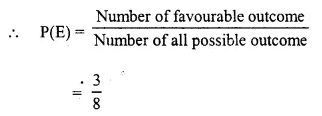(ii) At least two heads : These can be HHH,HHT, HTH, THH = 4 in numbers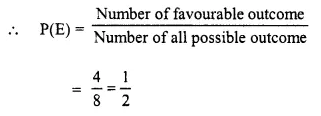(iii) Atmost two heads : These can be THH, HHT, HTH, HTT, THT, TTH, TTT which is 7 in numbers.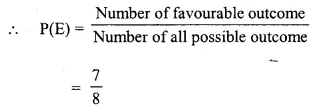(iv) All tails : There can be TTT i. e., only one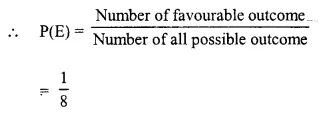(v) At least one tail : There can be HHT, HTH, HTT, TTT, THH, THT, TTH = 7 in number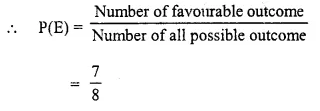Question 28.
Two dice are thrown simultaneously. What is the probability that :
(i) 4 will not come up either time ?
(ii) 4 will come up at least once ?
Solution:
Two dice are thrown simultaneous,
and each dice has 1-6 numbers on its faces
∴ Number of possible outcome = 6 x 6 = 36
(i) 4 will not come up either time
∴ Number of favourable outcomes = (6 – 1)² = (5)² = 25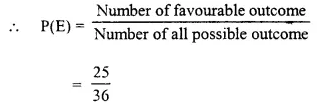(ii) 4 will come up at least once
∴ These can be (1, 4), (2, 4), (3, 4), (4, 4), (4, 5),(4, 6), (4, 1), (4, 2), (4, 3), (4, 5), (4, 6)
∴ Number of favourable outcome =11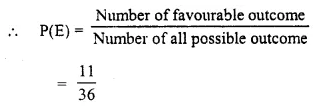Question 29.
Cards marked with numbers 1, 2, 3, 4, …,20 are well shuffled and a card is drawn at random. What is the probability that the number on the card is
(i) a prime number
(ii) divisible by 3
(iii) a perfect square ?
Solution: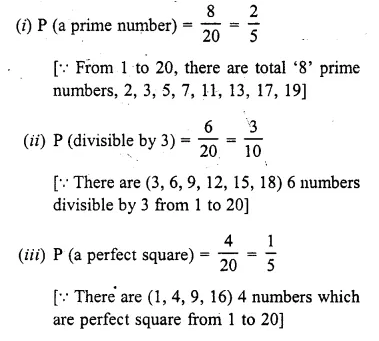Question 30.
Offices in Delhi are open for five days in a week (Monday to Friday). Two employees of an office remain absent for one day in the same particular week. Find the probability that they remain absent on :
(i) the same day
(ii) consecutive day
(iii) different days.
Solution:
∵ Office are open for 5 days a week
∴ Number of possible outcomes for 2 employees = 5 x 5 = 25
Let the five days of working be denoted by M, T, W, Th, F for Mondays, Tuesday, Wednesday Thursday and Friday respectively
(i) On Same day.
Favourable outcome will be M;T;T;M;T;W;W;T;W;H; TH.W ; TH ; F and F, TH which are 8 in all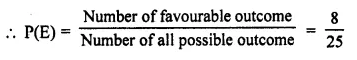(iii) Absent on different days : Then = 1- P(absent on the same day)
= 1 – $$\frac { 1 }{ 5 }$$= $$\frac { 4 }{ 5 }$$ [from (i)]
∵ P(E) + P(not E) = 1

Question 31.
A box contains some black balls and 30 white balls. If the probability of drawing a black ball is two-fifths of white ball; find the number of black balls in the box.
Solution:
In a box, some balls are black while 30 balls are white
Let number of black balls = x
Then number of possible outcome = x + 30
Probability of drawing a black ball = $$\frac { 2 }{ 5 }$$ of a white balls.
Now, In case of black ball,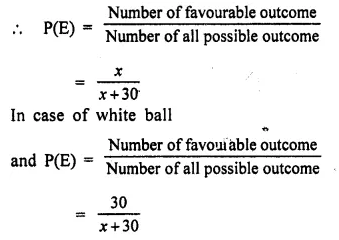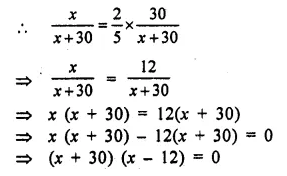Either x + 30 = 0, then x = -30 which is not possible
or x – 12 = 0, then x = 12
Hence number of black balls = 12

Question 32.
From a pack of 52 playing cards all cards whose numbers are multiples of 3 are removed. A card is now drawn at random.
What is the probability that the card drawn is
(i) a face card (King, Jack or Queen)
(ii) an even numbered red card ? (2011)
Solution:
No. of total cards = 52 cards removed of 4 colors = 3, 6, 9, 12 = 4 x 4 = 16
Remain using cards = 52 – 16 = 36
(i) No. of faces cards = 2 x 4 = 8 cards (excluding queen)
∴ Probebility P(E) = $$\frac { 8 }{ 36 }$$ = $$\frac { 2 }{ 9 }$$
(ii) An even number red cards = 2, 4, 8, 10 = 4×2 = 8 cards
∴ Probebility P(E) = $$\frac { 8 }{ 36 }$$ = $$\frac { 2 }{ 9 }$$

Question 33.
A die has 6 faces marked by the given numbers as shown below: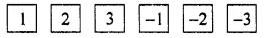The die is thrown once. What is the probability of getting
(i) a positive integer.
(ii) an integer greater than -3.
(iii) the smallest integer.
Solution:
Total outcomes n(S)= 6
(i) a positive integer = (1, 2, 3)
No. of favourables n(E) = 3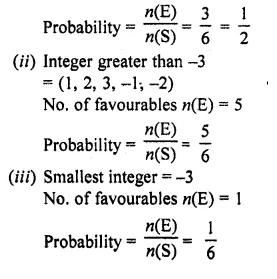Question 34.
A bag contains 5 white balls, 6 red balls and 9 green balls. A ball is drawn at random from the bag. Find the probability that the ball drawn is :
(i) a green ball.
(ii) a white or a red ball.
(iii) neither a green ball nor a white ball. (2015)
Solution:
A bag contains 5 white balls, 6 red balls and 9 green balls.
∴ Total number of balls = 5 + 6 + 9 = 20 balls
One ball is drawn at random.
(i) Probability of a green ball = $$\frac { 9 }{ 20 }$$
(ii) Probability of a white or a red ball = $$\frac { 5 + 6 }{ 20 }$$ = $$\frac { 11 }{ 20 }$$
(iii) Probability of neither a green ball nor a white ball = $$\frac { 6 }{ 20 }$$ = $$\frac { 3 }{ 10 }$$ (Only red balls )

Question 35.
A game of numbers has cards marked with 11, 12, 13, , 40. A card is drawn at random. Find the probability that the number on the card drawn is :
(i) A perfect square
(ii) Divisible by 7
Solution:
(i) The perfect squares lying between 11 and 40 and 16, 25 and 36.
So the number of possible outcomes is 3.
Total number of cards from 11 to 40 is 40 – 11 + 1 = 30
Probability that the number on the card drawn is a perfect square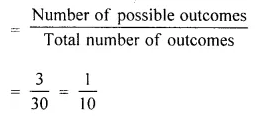So, the probability that the number on the
card drawn is a perfect square is $$\frac { 1 }{ 10 }$$ .
(ii) The numbers lfom 11 to 40 that are divisible by 7 are 14, 21, 28 and 35.
So the number of possible outcomes is 4. Total number of cards from 11 to 40 is 30.
Probability that the number on the card drawn is divisible by 7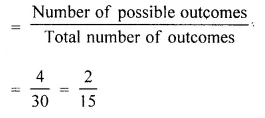So, the probability that the number on the card drawn is divisible by 7 is $$\frac { 2 }{ 15 }$$.

Hope given Selina Concise Mathematics Class 10 ICSE Solutions Chapter 25 Probability Ex 25C are helpful to complete your math homework.

If you have any doubts, please comment below. Learn Insta try to provide online math tutoring for you.